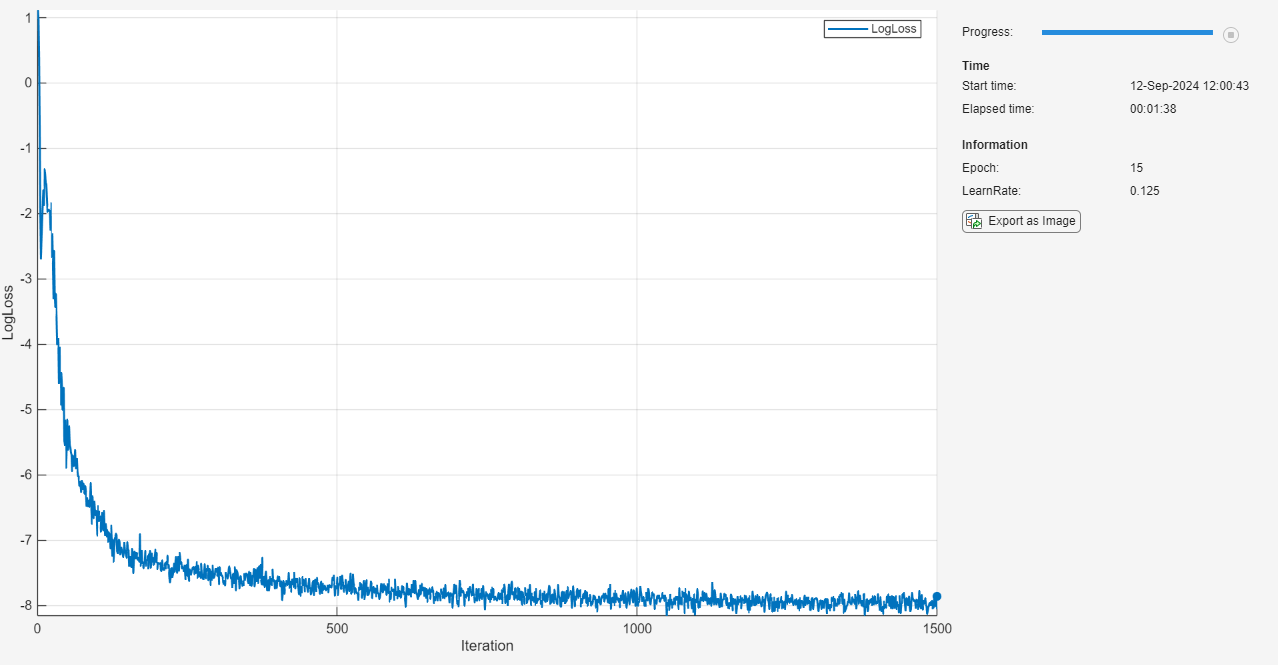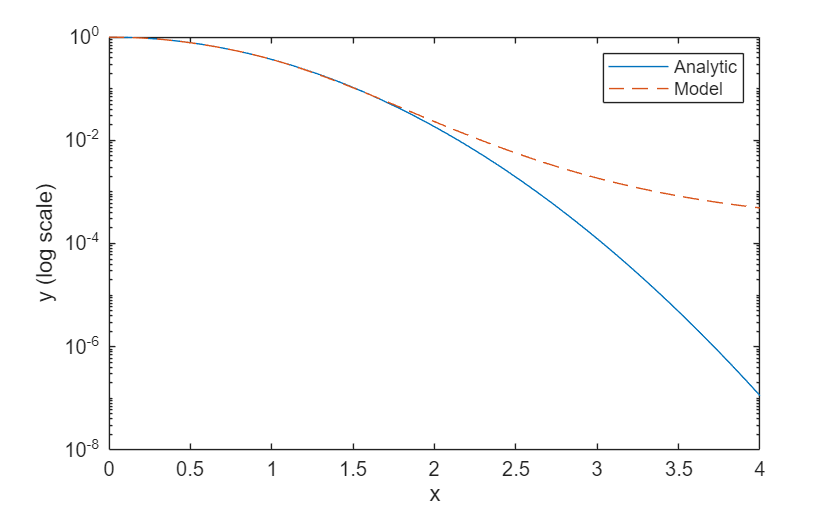# Solve Ordinary Differential Equation Using Neural Network

This example shows how to solve an ordinary differential equation (ODE) using a neural network.

Not all differential equations have a closed-form solution. To find approximate solutions to these types of equations, many traditional numerical algorithms are available. However, you can also solve an ODE by using a neural network. This approach comes with several advantages, including that it provides differentiable approximate solutions in a closed analytic form .

This example shows you how to:

1. Generate training data in the range $x\in \left[0,2\right]$.

2. Define a neural network that takes $x$ as input and returns the approximate solution to the ODE $\stackrel{˙}{\text{\hspace{0.17em}}\mathit{y}}=-2\mathit{xy}$, evaluated at $x$, as output.

3. Train the network with a custom loss function.

4. Compare the network predictions with the analytic solution.

### ODE and Loss Function

In this example, you solve the ODE

`$\stackrel{˙}{\text{\hspace{0.17em}}\mathit{y}}=-2\mathit{xy},$`

with the initial condition $\mathit{y}\left(0\right)=1$. This ODE has the analytic solution

`$\mathit{y}\left(\mathit{x}\right)={\mathit{e}}^{-{\mathit{x}}^{2}}.$`

Define a custom loss function that penalizes deviations from satisfying the ODE and the initial condition. In this example, the loss function is a weighted sum of the ODE loss and the initial condition loss:

`${L}_{\theta }\left(x\right)=‖{\underset{}{\overset{˙}{y}}}_{\theta }+2x{y}_{\theta }{‖}^{2}+k‖{y}_{\theta }\left(0\right)-1{‖}^{2}$`

$\theta \text{\hspace{0.17em}}$is the network parameters, $k$ is a constant coefficient, ${\mathit{y}}_{\theta \text{\hspace{0.17em}}}$ is the solution predicted by the network, and $\stackrel{˙}{{\mathit{y}}_{\theta \text{\hspace{0.17em}}}}$ is the derivative of the predicted solution computed using automatic differentiation. The term $‖\text{\hspace{0.17em}}\stackrel{˙}{{\mathit{y}}_{\theta \text{\hspace{0.17em}}}}\text{\hspace{0.17em}}+\text{\hspace{0.17em}}2{\mathit{xy}}_{\theta \text{\hspace{0.17em}}}\text{\hspace{0.17em}}{‖}^{2}$ is the ODE loss and it quantifies how much the predicted solution deviates from satisfying the ODE definition. The term $‖{\mathit{y}}_{\theta \text{\hspace{0.17em}}}\left(0\right)-1\text{\hspace{0.17em}}{‖}^{2}$ is the initial condition loss and it quantifies how much the predicted solution deviates from satisfying the initial condition.

### Generate Input Data and Define Network

Generate 10,000 training data points in the range $x\in \left[0,2\right]$.

`x = linspace(0,2,10000)';`

Define the network for solving the ODE. As the input is a real number $x\in \mathbb{R}$, specify an input size of 1.

```inputSize = 1; layers = [ featureInputLayer(inputSize,Normalization="none") fullyConnectedLayer(10) sigmoidLayer fullyConnectedLayer(1) sigmoidLayer];```

Create a `dlnetwork` object from the layer array.

`dlnet = dlnetwork(layers)`
```dlnet = dlnetwork with properties: Layers: [5×1 nnet.cnn.layer.Layer] Connections: [4×2 table] Learnables: [4×3 table] State: [0×3 table] InputNames: {'input'} OutputNames: {'layer_2'} Initialized: 1 ```

### Define Model Gradients Function

Create the function `modelGradients`, listed at the end of the example, which takes as inputs a `dlnetwork` object `dlnet`, a mini-batch of input data `dlX`, and the coefficient associated with the initial condition loss `icCoeff`. This function returns the gradients of the loss with respect to the learnable parameters in `dlnet` and the corresponding loss.

### Specify Training Options

Train for 15 epochs with a mini-batch size of 100.

```numEpochs = 15; miniBatchSize = 100;```

Specify the options for SGDM optimization. Specify a learning rate of 0.5, a learning rate drop factor of 0.5, a learning rate drop period of 5, and a momentum of 0.9.

```initialLearnRate = 0.5; learnRateDropFactor = 0.5; learnRateDropPeriod = 5; momentum = 0.9;```

Specify the coefficient of the initial condition term in the loss function as 7. This coefficient specifies the relative contribution of the initial condition to the loss. Tweaking this parameter can help training converge faster.

`icCoeff = 7;`

### Train Model

To use mini-batches of data during training:

1. Create an `arrayDatastore` object from the training data.

2. Create a `minibatchqueue` object that takes the `arrayDatastore` object as input, specify a mini-batch size, and format the training data with the dimension labels `'BC'` (batch, channel).

```ads = arrayDatastore(x,IterationDimension=1); mbq = minibatchqueue(ads,MiniBatchSize=miniBatchSize,MiniBatchFormat="BC");```

By default, the `minibatchqueue` object converts the data to `dlarray` objects with underlying type `single`.

Train on a GPU if one is available. By default, the `minibatchqueue` object converts each output to a `gpuArray` if a GPU is available. Using a GPU requires Parallel Computing Toolbox™ and a supported GPU device. For information on supported devices, see GPU Computing Requirements (Parallel Computing Toolbox).

Initialize the training progress plot.

```figure set(gca,YScale="log") lineLossTrain = animatedline(Color=[0.85 0.325 0.098]); ylim([0 inf]) xlabel("Iteration") ylabel("Loss (log scale)") grid on```

Initialize the velocity parameter for the SGDM solver.

`velocity = [];`

Train the network using a custom training loop. For each epoch, shuffle the data and loop over mini-batches of data. For each mini-batch:

• Evaluate the model gradients and loss using the `dlfeval` and `modelGradients` functions.

• Update the network parameters using the `sgdmupdate` function.

• Display the training progress.

Every `learnRateDropPeriod` epochs, multiply the learning rate by `learnRateDropFactor`.

```iteration = 0; learnRate = initialLearnRate; start = tic; % Loop over epochs. for epoch = 1:numEpochs % Shuffle data. mbq.shuffle % Loop over mini-batches. while hasdata(mbq) iteration = iteration + 1; % Read mini-batch of data. dlX = next(mbq); % Evaluate the model gradients and loss using dlfeval and the modelGradients function. [gradients,loss] = dlfeval(@modelGradients, dlnet, dlX, icCoeff); % Update network parameters using the SGDM optimizer. [dlnet,velocity] = sgdmupdate(dlnet,gradients,velocity,learnRate,momentum); % To plot, convert the loss to double. loss = double(gather(extractdata(loss))); % Display the training progress. D = duration(0,0,toc(start),Format="mm:ss.SS"); addpoints(lineLossTrain,iteration,loss) title("Epoch: " + epoch + " of " + numEpochs + ", Elapsed: " + string(D)) drawnow end % Reduce the learning rate. if mod(epoch,learnRateDropPeriod)==0 learnRate = learnRate*learnRateDropFactor; end end```### Test Model

Test the accuracy of the network by comparing its predictions with the analytic solution.

Generate test data in the range $x\in \left[0,4\right]$ to see if the network is able to extrapolate outside the training range $x\in \left[0,2\right]$.

`xTest = linspace(0,4,1000)';`

To use mini-batches of data during testing:

1. Create an `arrayDatastore` object from the testing data.

2. Create a `minibatchqueue` object that takes the `arrayDatastore` object as input, specify a mini-batch size of 100, and format the training data with the dimension labels `'BC'` (batch, channel).

```adsTest = arrayDatastore(xTest,IterationDimension=1); mbqTest = minibatchqueue(adsTest,MiniBatchSize=100,MiniBatchFormat="BC");```

Loop over the mini-batches and make predictions using the `modelPredictions` function, listed at the end of the example.

`yModel = modelPredictions(dlnet,mbqTest);`

Evaluate the analytic solution.

`yAnalytic = exp(-xTest.^2);`

Compare the analytic solution and the model prediction by plotting them on a logarithmic scale.

```figure; plot(xTest,yAnalytic,"-") hold on plot(xTest,yModel,"--") legend("Analytic","Model") xlabel("x") ylabel("y (log scale)") set(gca,YScale="log")```The model approximates the analytic solution accurately in the training range $x\in \left[0,2\right]$ and it extrapolates in the range $x\in \left(2,4\right]$ with lower accuracy.

Calculate the mean squared relative error in the training range $x\in \left[0,2\right]$.

```yModelTrain = yModel(1:500); yAnalyticTrain = yAnalytic(1:500); errorTrain = mean(((yModelTrain-yAnalyticTrain)./yAnalyticTrain).^2) ```
```errorTrain = single 4.3454e-04 ```

Calculate the mean squared relative error in the extrapolated range $x\in \left(2,4\right]$.

```yModelExtra = yModel(501:1000); yAnalyticExtra = yAnalytic(501:1000); errorExtra = mean(((yModelExtra-yAnalyticExtra)./yAnalyticExtra).^2) ```
```errorExtra = single 17576612 ```

Notice that the mean squared relative error is higher in the extrapolated range than it is in the training range.

### Model Gradients Function

The `modelGradients` function takes as inputs a `dlnetwork` object `dlnet`, a mini-batch of input data `dlX`, and the coefficient associated with the initial condition loss `icCoeff`. This function returns the gradients of the loss with respect to the learnable parameters in `dlnet` and the corresponding loss. The loss is defined as a weighted sum of the ODE loss and the initial condition loss. The evaluation of this loss requires second order derivatives. To enable second order automatic differentiation, use the function `dlgradient` and set the `EnableHigherDerivatives` name-value argument to `true`.

```function [gradients,loss] = modelGradients(dlnet, dlX, icCoeff) y = forward(dlnet,dlX); % Evaluate the gradient of y with respect to x. % Since another derivative will be taken, set EnableHigherDerivatives to true. dy = dlgradient(sum(y,"all"),dlX,EnableHigherDerivatives=true); % Define ODE loss. eq = dy + 2*y.*dlX; % Define initial condition loss. ic = forward(dlnet,dlarray(0,"CB")) - 1; % Specify the loss as a weighted sum of the ODE loss and the initial condition loss. loss = mean(eq.^2,"all") + icCoeff * ic.^2; % Evaluate model gradients. gradients = dlgradient(loss, dlnet.Learnables); end```

### Model Predictions Function

The `modelPredictions` function takes a `dlnetwork` object `dlnet` and a `minibatchqueue` of input data `mbq` and computes the model predictions `y` by iterating over all data in the `minibatchqueue` object.

```function Y = modelPredictions(dlnet,mbq) Y = []; while hasdata(mbq) % Read mini-batch of data. dlXTest = next(mbq); % Predict output using trained network. dlY = predict(dlnet,dlXTest); YPred = gather(extractdata(dlY)); Y = [Y; YPred']; end end```

### References

1. Lagaris, I. E., A. Likas, and D. I. Fotiadis. “Artificial Neural Networks for Solving Ordinary and Partial Differential Equations.” IEEE Transactions on Neural Networks 9, no. 5 (September 1998): 987–1000. https://doi.org/10.1109/72.712178.